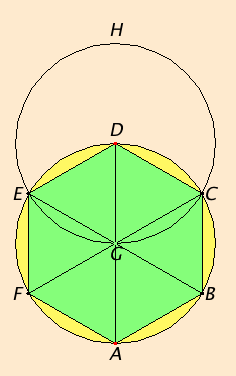# Proposition 15

To inscribe an equilateral and equiangular hexagon in a given circle.

Let ABCDEF be the given circle.

It is required to inscribe an equilateral and equiangular hexagon in the circle ABCDEF.

III.1

Draw the diameter AD of the circle ABCDEF. Take the center G of the circle. Describe the circle EGCH with center D and radius DG. Join EG and CG and carry them through to the points B and F. Join AB, BC, CD, DE, EF, and FA.

I say that the hexagon ABCDEF is equilateral and equiangular.For, since the point G is the center of the circle ABCDEF, GE equals GD.

Again, since the point D is the center of the circle GCH, DE equals DG.

I.5

But GE was proved equal to GD, therefore GE also equals ED. Therefore the triangle EGD is equilateral, and therefore its three angles EGD, GDE, and DEG equal one another, inasmuch as, in isosceles triangles, the angles at the base equal one another.

I.32

And the sum of the three angles of the triangle equals two right angles, therefore the angle EGD is one-third of two right angles.

Similarly, the angle DGC can also be proved to be one third of two right angles.

I.13

And, since the straight line CG standing on EB makes the sum of the adjacent angles EGC and CGB equal to two right angles, therefore the remaining angle CGB is also one-third of two right angles.

I.15

Therefore the angles EGD, DGC, and CGB equal one another, so that the angles vertical to them, the angles BGA, AGF, and FGE, are equal.

Therefore the six angles EGD, DGC, CGB, BGA, AGF, and FGE equal one another.

III.26

But equal angles stand on equal circumferences, therefore the six circumferences AB, BC, CD, DE, EF, and FA equal one another.

III.29

And straight lines that cut off equal circumferences are equal, therefore the six straight lines equal one another. Therefore the hexagon ABCDEF is equilateral.

I say next that it is also equiangular.

III.27

For, since the circumference FA equals the circumference ED, add the circumference ABCD to each, therefore the whole FABCD equals the whole EDCBA. And the angle FED stands on the circumference FABCD, and the angle AFE on the circumference EDCBA, therefore the angle AFE equals the angle DEF.

Similarly it can be proved that the remaining angles of the hexagon ABCDEF are also severally equal to each of the angles AFE and FED, therefore the hexagon ABCDEF is equiangular.

But it was also proved equilateral, and it has been inscribed in the circle ABCDEF.

Therefore an equilateral and equiangular hexagon has been inscribed in the given circle.

Q.E.F.

# Corollary

From this it is clear that he side of the hexagon equals the radius of the circle.

And, in like manner as in the case of the pentagon, if through the points of division on the circle we draw tangents to the circle, there will be circumscribed about the circle an equilateral and equiangular hexagon in conformity with what was explained in the case of the pentagon.

And further by means similar to those explained in the case of the pentagon we can both inscribe a circle in a given hexagon and circumscribe one about it.

## Guide

The corollary is used in several propositions in Book XIII starting with
XIII.9.